## RS Aggarwal Class 6 Solutions Chapter 8 Algebraic Expressions Ex 8A

These Solutions are part of RS Aggarwal Solutions Class 6. Here we have given RS Aggarwal Solutions Class 6 Chapter 8 Algebraic Expressions Ex 8A.

Other Exercises

Question 1.
Solution:
(i) x + 12
(ii) y – 7
(iii) a – b
(iv) (x + y) + xy
(v) $$\\ \frac { 1 }{ 3 }$$x (a + b)
(vi) 7y + 5x
(vii) $$x+ \frac { y }{ 5 }$$
(viii) 4 – x
(ix) $$\\ \frac { x }{ y } -2$$
(x) x2
(xi) 2x + y
(xii) y2 + 3x
(xiii) x – 2y
(xiv) y3 – x3
(xv) $$\\ \frac { x }{ 8 } \times y$$

Question 2.
Solution:
Marks scored in English = 80
Marks scored in Hindi = x
∴ Total score in the two subjects = 80 + x

Question 3.
Solution:
We can write :
(i) b × b × b ×….15 times = 615
(ii) y × y × y ×…..20 times = y20
(iii) 14 × a × a × a × a × b × b × b= 14a4 b3
(iv) 6 × x × x × y × y = 6x2y2
(v) 3 × z × z × z × y × y × x= 3z3y2x

Question 4.
Solution:
We can write :
(i) x2y4 = x × x × y × y × y × y
(ii) 6y5 = 6 × y × y × y × y × y
(iii) 9xy2z = 9 × x × y × y × z
(iv) 10a3b3c3 = 10 × a × a × a × b × b × b × c × c × c

Hope given RS Aggarwal Solutions Class 6 Chapter 8 Algebraic Expressions Ex 8A are helpful to complete your math homework.

If you have any doubts, please comment below. Learn Insta try to provide online math tutoring for you.

## RS Aggarwal Class 6 Solutions Chapter 7 Decimals Ex 7E

These Solutions are part of RS Aggarwal Solutions Class 6. Here we have given RS Aggarwal Solutions Class 6 Chapter 7 Decimals Ex 7E.

Other Exercises

Question 1.
Solution:
(c) $$\\ \frac { 7 }{ 10 }$$ = 0.7

Question 2.
Solution:
(d) $$\\ \frac { 5 }{ 100 }$$ = .05

Question 3.
Solution:
(b) $$\\ \frac { 9 }{ 1000 }$$ = 0.009

Question 4.
Solution:
(a) $$\\ \frac { 16 }{ 1000 }$$ = 0.016

Question 5.
Solution:
(c) $$\\ \frac { 134 }{ 1000 }$$ = 0.134

Question 6.
Solution:
(a) $$2 \frac { 17 }{ 100 }$$ = 2.17

Question 7.
Solution:
(b) $$4 \frac { 3 }{ 1000 }$$ = 4.03

Question 8.
Solution:
(b) 6.25 = $$6 \frac { 25 }{ 100 }$$ = $$6 \frac { 1 }{ 4 }$$

Question 9.
Solution:
(b) $$\\ \frac { 6 }{ 25 }$$
= $$\\ \frac { 6\times 4 }{ 25\times 4 }$$
= $$\\ \frac { 24 }{ 100 }$$
= 0.24

Question 10.
Solution:
(c) $$4 \frac { 7 }{ 8 }$$ = $$\\ \frac { 39 }{ 8 }$$ = 4.875

Question 11.
Solution:
(a) 24.8 = $$24 \frac { 8 }{ 10 }$$
= $$24 \frac { 4 }{ 5 }$$

Question 12.
Solution:
(b) $$2 \frac { 1 }{ 25 }$$
= 2 + $$\\ \frac { 1 }{ 25 }$$ x $$\\ \frac { 4 }{ 4 }$$
= 2 + $$\\ \frac { 4 }{ 100 }$$
= 2.04

Question 13.
Solution:
(c) 2 + $$\\ \frac { 3 }{ 10 }$$ + $$\\ \frac { 4 }{ 100 }$$
= 2 + $$\\ \frac { 30 }{ 100 }$$ + $$\\ \frac { 4 }{ 100 }$$
= 2.34

Question 14.
Solution:
(b) $$2 \frac { 6 }{ 100 }$$
= 2 + 0.06
= 2.06

Question 15.
Solution:
(c) $$\\ \frac { 4 }{ 100 }$$ + $$\\ \frac { 7 }{ 10000 }$$
= 0.04 + 0.0007
= 0.0407

Question 16.
Solution:
(c) 2.06
= $$\left( 2\times 1 \right) +\left( 6\times \frac { 1 }{ 100 } \right)$$
= $$2+\frac { 6 }{ 100 }$$

Question 17.
Solution:
(d) Among 2.600, 2.006, 2.660,2.080, 2.660 is the largest.

Question 18.
Solution:
(b) 2.002 < 2.020 < 2.200 < 2.222 is the correct.

Question 19.
Solution:
(a) 2.1 = 2.100 and 2.005
2.100 > 2.055
=> 2.1 > 2.055

Question 20.
Solution:
(b) 1cm = $$\\ \frac { 1 }{ 100 }$$ m
= 0.01

Question 21.
Solution:
(b) 2 m 5 cm = 2.05 m

Question 22.
Solution:
(c) 2 kg 8 g = 2 + 0.008 = 2.008

Question 23.
Solution:
(b) 2 kg 56 g = 2.056 kg
(∵ 1000 g = 1 kg)

Question 24.
Solution:
(c) 2 km 35 m = 2.035 km
(∵ 1000 m = 1 km)

Question 25.
Solution:
(c) ∵ 0.4 + 0.004 + 4.4
= 4.804

Question 26.
Solution:
(a) ∵ 3.5 + 4.05 – 6.005
= 3.500 + 4.050 – 6.005
= 7.550 – 6.005
= 1.545

Question 27.
Solution:
(b) ∵6.3 – 2.8 = 3.5

Question 28.
Solution:
(c) ∵ 5.01 – 3.6 = 5.01 – 3.60
= 1.41

Question 29.
Solution:
(a) ∵ 2 – 0.7 = 2.0 – 0.7 = 1.3

Question 30.
Solution:
(a) ∵ 1.1 – 0.3
= 0.8

Hope given RS Aggarwal Solutions Class 6 Chapter 7 Decimals Ex 7E are helpful to complete your math homework.

If you have any doubts, please comment below. Learn Insta try to provide online math tutoring for you.

## RS Aggarwal Class 6 Solutions Chapter 7 Decimals Ex 7D

These Solutions are part of RS Aggarwal Solutions Class 6. Here we have given RS Aggarwal Solutions Class 6 Chapter 7 Decimals Ex 7D.

Other Exercises

Question 1.
Solution:
27.86 from 53.74
= 53.74 – 27.86
= 25.88 Ans.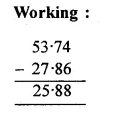Question 2.
Solution:
64.98 from 103.87
103.87 – 64.98
= 38.89 Ans.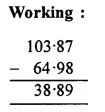Question 3.
Solution:
59.63 from 92.4
92.40 – 59.63
= 32.77 Ans.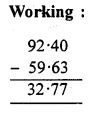Question 4.
Solution:
56.8 from 204
204.0 – 56.8
= 147.2 Ans.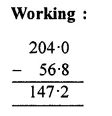Question 5.
Solution:
127.38 from 216.2
216.20 – 127.38
= 88.82 Ans.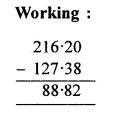Question 6.
Solution:
39.875 from 70.68
70.680 – 39.875
= 30.805 Ans.Question 7.
Solution:
523.120 – 348.237
= 174.883 Ans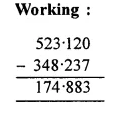Question 8.
Solution:
600.000 – 458.573
= 141.427 Ans.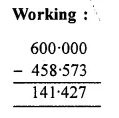Question 9.
Solution:
206.321 – 149.456
= 56.865 Ans.Question 10.
Solution:
3.400 – 0.612
= 2.788 Ans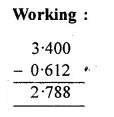Question 11.
Solution:
Converting them in like decimals
37.600 + 72.850 – 58.678 – 6.090
= (37.600 + 72.850) – (58.678 + 6.090)
= 110.450 – 64.768
= 45.682Question 12.
Solution:
75.3 – 104.645 + 178.96 – 47.9
= 75.300 – 104.645 + 178.960 – 47.900
(Converting into like decimals)
= 75.300 + 178.960 – 104.645 – 47.900
= (75.300 + 178.960) – (104.645 + 47.900)
= 254.260 – 152.545
= 101.715 Ans.Question 13.
Solution:
213.4 – 56.84 – 11.87 – 16.087
= 213.400 – 56.840 – 11.870 – 16.087
(Converting into like decimals)
= 213.400 – (56.840 + 11.870 + 16.087)
= 213.400 – 84.797
= 128.603 Ans.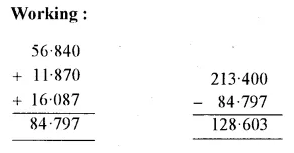Question 14.
Solution: 76.3 . 7.666 . 6.77
= 76.300 – 7.666 – 6.770
(Converting into like decimals)
= 76.300 – 14.436
= 61.864 Ans.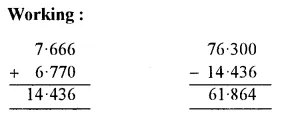Question 15.
Solution:
In order to get the required number, we have to subtract 74.5 from 91.
Required number = 91 – 74.5
= 91.0 – 74.5
= 16.5 Ans.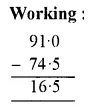Question 16.
Solution:
In order to get the required numbers, we have to subtract 0.862 from 7.3.
Required number = 7.3 – 0.862
= 7.300 – 0.862
= 6.438 Ans.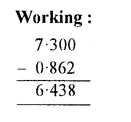Question 17.
Solution:
In order to get the required number, we have to subtract 23.754 from 50
Required number = 50 – 23.754
= 50.000 – 23.754
= 26.246 Ans.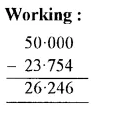Question 18.
Solution:
In order to get the required number, we should subtract 27.84 from 84.5
Required number = 84.5 – 27.84
= 84.50 – 27.84
= 56.66 Ans.Question 19.
Solution:
Weight of Neelam’s bag = 6 kg 80 g
Weight of Garima bag = 5 kg 265 g
Difference in their weights = 6 kg 80 g – 5 kg 265 g
= 6.080 kg – 5.265 kg
= 0.815 kg
= 815 g
Neelam’s bag is heavier by 815 g Ans.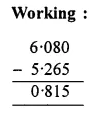Question 20.
Solution:
Cost of a notebook = Rs. 19.75
Cost of a pencil = Rs. 3 .85
Cost of a pen = Rs. 8.35
Total cost = Rs. 19.75 + Rs. 3.85 + Rs. 8.35
= Rs. 31.95
Amount given to the bookshop = Rs. 50
Balance amount to get back = Rs. 50.00 – Rs. 31.95
= Rs. 18.05 Ans.Question 21.
Solution:
Weight of fruits = 5 kg 75 g .
Weight of vegetables = 3 kg 465 kg
Total weight of both = 5 kg 75 g + 3 kg 465 g
= 5.075 kg + 3.465 kg
= 8.540 kg
Gross weight of bag with these things = 9 kg
Net weight of bag = 9.000 – 8.540
= 0.460 kg
= 460 g Ans.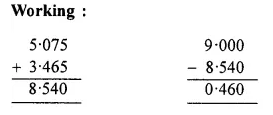Question 22.
Solution:
Total distance = 14 km
Distance covered by scooter = 10 km 65 m
Distance covered by bus = 3 km 75 m
Total distance covered by scooter and by bus = 10 km 65 m + 3 km 75 m
= 10.065 km + 3 075 m
= 13.140 km
Remaining distance covered by walking
= (14.000 – 13.140) km
= 0.860 km
= 860 m Ans.Hope given RS Aggarwal Solutions Class 6 Chapter 7 Decimals Ex 7D are helpful to complete your math homework.

If you have any doubts, please comment below. Learn Insta try to provide online math tutoring for you.

## RS Aggarwal Class 6 Solutions Chapter 7 Decimals Ex 7C

These Solutions are part of RS Aggarwal Solutions Class 6. Here we have given RS Aggarwal Solutions Class 6 Chapter 7 Decimals Ex 7C.

Other Exercises

Question 1.
Solution:
9.6, 14.8, 37 and 5.9
Converting these decimals into like decimals and then adding 9.6 + 14.8 + 37.0 + 5.9
= 67.3 Ans.
Working :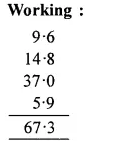Question 2.
Solution:
23.7, 106.94, 68.9 and 29.5
Converting them into like decimals and then adding
23.70 + 106.94 + 68.90 + 29.50
= 229.04 Ans.
Working :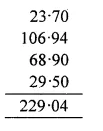Question 3.
Solution:
72.8, 7.68, 16.23 and 0.7
Converting them into like decimals and then adding
72.80 + 7.68 + 16.23 + 0.70
= 97.41 Ans.
Working :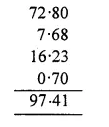Question 4.
Solution:
18.6, 84.75, 8.345 and 9.7
Converting them into like decimals and then adding
18.600 + 84.750 + 8.345 + 9.700
= 121.395 Ans.
Working :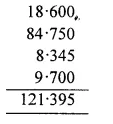Question 5.
Solution:
8.236, 16.064, 63.8 and 27.53
Converting them into like decimals and then adding
8.236 + 16.064 + 63.800 + 27.530
= 115.630 Ans.
Working :Question 6.
Solution:
28.9, 19.64, 123.697 and 0.354
Converting them into like decimals and then adding
28.900 + 19.640 + 123.697 + 0.354
= 172.591 Ans.
Working :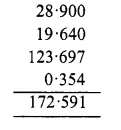Question 7.
Solution:
4.37, 9.638, 17.007 and 6.8
Converting them into like decimals and then adding
4. 370 + 9.638 + 17.007 + 6.800
= 37.815 Ans.
Working :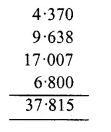Question 8.
Solution:
14.5, 0.038, 118.573 and 6.84
Converting them into like decimals and then adding
14.500 + 0.038 + 118.573 + 6.840
= 139.951 Ans.
Working :Question 9.
Solution:
Earning for the first day = 32.60 rupees
Earning for the second day = 56.80 rupees
Earning for the third day = 72 rupees
Total earning = Rs. 32.60 + Rs. 56.80 + Rs. 72
= Rs. 161.40 Ans.
WorkingQuestion 10.
Solution:
Cost of almirah = Rs. 11025
Cartage = Rs. 172.50
Cost on repair = Rs. 64.80
Total cost = Rs. 11025 + Rs. 172.50 + Rs. 64.80
= Rs. 11262.30 Ans.
Working :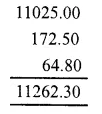Question 11.
Solution:
Distance covered by taxi = 36 km 235 m
= 36.235 km
Distance covered by Rickshaw = 4 km 85 m
= 4.085 km
and distance covered on foot
= 1 km 80 m
= 1.080 m
Total distance covered = 36.235 km + 4.085 km + 1.080 km
= 41.400 km
= 41 km 400 m Ans.
Working :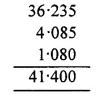Question 12.
Solution:
Weight of sugar in a bag = 45 kg 80 g
= 45.080 kg
Mass (weight) of empty bag = 950 g
= 0.950 kg
Total weight of the bag with sugar = 45 kg 80 g + 950 g
= 45.080 kg + 0.950 kg
= 46.030 kg
= 46 kg 30 g Ans.
Working :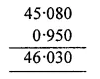Question 13.
Solution:
Length of cloth for shirt = 2 m 70 cm
= 2.70 m
Length of cloth for pyjamas = 2 m 60 cm
= 2.60 m
Total length of cloth = 2.70 m + 2.60 m
= 5.30 m
= 5 m 30 cm Ans.
Working :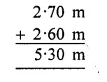Question 14.
Solution:
Cloth of salwar = 2 m 5 cm = 2.05 m
Cloth for shirt = 3 m 35 cm = 3.35 m
Total length of cloth = 2.05 m + 3.35 m
= 5.4.0 m
= 5 m 40 cm Ans.
Working :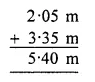Hope given RS Aggarwal Solutions Class 6 Chapter 7 Decimals Ex 7C are helpful to complete your math homework.

If you have any doubts, please comment below. Learn Insta try to provide online math tutoring for you.

## RS Aggarwal Class 6 Solutions Chapter 7 Decimals Ex 7B

These Solutions are part of RS Aggarwal Solutions Class 6. Here we have given RS Aggarwal Solutions Class 6 Chapter 7 Decimals Ex 7B.

Other Exercises

Convert each of the following into a fraction in its simplest form :

Question 1.
Solution:
.9 = $$\\ \frac { 9 }{ 10 }$$

Question 2.
Solution:
0.6
= $$\\ \frac { 6 }{ 10 }$$
= $$\frac { 6\div 2 }{ 10\div 2 }$$
= $$\\ \frac { 3 }{ 5 }$$
(Dividing by 2, the HCF of 6, 10)

Question 3.
Solution:
.08
= $$\\ \frac { 8 }{ 100 }$$
= $$\frac { 8\div 4 }{ 100\div 4 }$$
= $$\\ \frac { 2 }{ 25 }$$
(Dividing by 4, the HCF of 7, 100)

Question 4.
Solution:
0.15
= $$\\ \frac { 15 }{ 100 }$$
= $$\frac { 15\div 5 }{ 100\div 5 }$$
= $$\\ \frac { 3 }{ 20 }$$
(Dividing by 5, the HCF of 15, 100)

Question 5.
Solution:
0.48
= $$\\ \frac { 48 }{ 100 }$$
= $$\frac { 48\div 4 }{ 100\div 4 }$$
= $$\\ \frac { 12 }{ 25 }$$
(Dividing by 4, the HCF of 48, 100)

Question 6.
Solution:
0.53
= $$\\ \frac { 53 }{ 1000 }$$

Question 7.
Solution:
= $$\\ \frac { 125 }{ 1000 }$$
= $$\frac { 125\div 125 }{ 1000\div 125 }$$
= $$\\ \frac { 1 }{ 8 }$$
(Dividing by 125, the HCF of 125, 1000)

Question 8.
Solution:
.224
= $$\\ \frac { 224 }{ 1000 }$$
= $$\frac { 224\div 8 }{ 1000\div 8 }$$
= $$\\ \frac { 28 }{ 125 }$$
(Dividing by 8, the HCF of 224, 1000)

Convert each of the following as a mixed fraction

Question 9.
Solution:
6.4
= $$\\ \frac { 64 }{ 10 }$$
= $$\frac { 64\div 2 }{ 10\div 2 }$$
= $$\\ \frac { 32 }{ 6 }$$
= $$6 \frac { 2 }{ 5 }$$
(Dividing by 2, the HCF of 64, 10)

Question 10.
Solution:
16.5
= $$\\ \frac { 165 }{ 10 }$$
= $$\frac { 165\div 5 }{ 10\div 5 }$$
= $$\\ \frac { 33 }{ 2 }$$
= $$16 \frac { 1 }{ 2 }$$
(Dividing by 5, the HCF of 165, 10)

Question 11.
Solution:
8.36
= $$\\ \frac { 836 }{ 100 }$$
= $$\frac { 836\div 4 }{ 100\div 4 }$$
= $$\\ \frac { 209 }{ 25 }$$
= $$8 \frac { 9 }{ 25 }$$
(Dividing by 4, the HCF of 836, 100)

Question 12.
Solution:
4.275
= $$\\ \frac { 4275 }{ 1000 }$$
= $$\frac { 4275\div 25 }{ 1000\div 25 }$$
= $$\\ \frac { 171 }{ 40 }$$
= $$4 \frac { 11 }{ 40 }$$
(Dividing by 25 )

Question 13.
Solution:
25.06
= $$\\ \frac { 2506 }{ 100 }$$
= $$\frac { 2506\div 2 }{ 100\div 2 }$$
= $$\\ \frac { 1253 }{ 50 }$$
= $$25 \frac { 3 }{ 50 }$$
(Dividing by 2 )

Question 14.
Solution:
7.004
= $$\\ \frac { 7004 }{ 1000 }$$
= $$\frac { 7004\div 4 }{ 1000\div 4 }$$
= $$\\ \frac { 1751 }{ 250 }$$
= $$7 \frac { 1 }{ 250 }$$
(Dividing by 4)

Question 15.
Solution:
2.052
= $$\\ \frac { 2052 }{ 1000 }$$
= $$\frac { 2052\div 4 }{ 1000\div 4 }$$
= $$\\ \frac { 513 }{ 250 }$$
= $$2 \frac { 13 }{ 250 }$$
(Dividing by 4)

Question 16.
Solution:
3.108
= $$\\ \frac { 3108 }{ 1000 }$$
= $$\frac { 3108\div 4 }{ 1000\div 4 }$$
= $$\\ \frac { 777 }{ 250 }$$
= $$3 \frac { 27 }{ 250 }$$
(Dividing by 4)

Question 17.
Solution:
$$\\ \frac { 23 }{ 10 }$$
= 2.3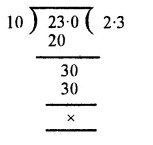Question 18.
Solution:
$$\\ \frac { 167 }{ 100 }$$
= 1.67Question 19.
Solution:
$$\\ \frac { 1589 }{ 100 }$$
= 15.89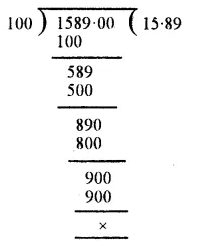Question 20.
Solution:
$$\\ \frac { 5413 }{ 1000 }$$
= 5.413Question 21.
Solution:
$$\\ \frac { 21415 }{ 1000 }$$
= 21.415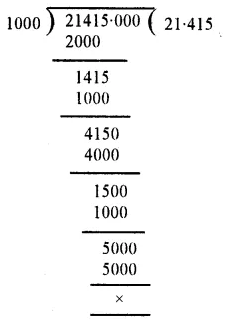Question 22.
Solution:
$$\\ \frac { 25 }{ 4 }$$
= 6.25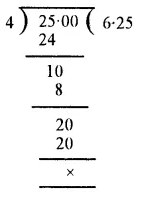Question 23.
Solution:
$$3 \frac { 3 }{ 5 }$$
= $$\\ \frac { 3\times 5+3 }{ 5 }$$
= $$\\ \frac { 15+3 }{ 5 }$$
= $$\\ \frac { 18 }{ 5 }$$
= 3.6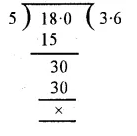Question 24.
Solution:
$$1 \frac { 4 }{ 25 }$$
= $$\\ \frac { 1\times 25+4 }{ 25 }$$
= $$\\ \frac { 25+4 }{ 25 }$$
= $$\\ \frac { 29 }{ 25 }$$
= 1.16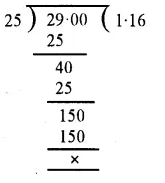Question 25.
Solution:
$$5 \frac { 17 }{ 50 }$$
= $$\\ \frac { 5\times 50+17 }{ 50 }$$
= $$\\ \frac { 250+17 }{ 50 }$$
= $$\\ \frac { 267 }{ 50 }$$
= 5.34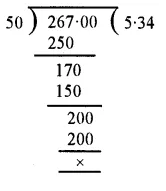Question 26.
Solution:
$$12 \frac { 3 }{ 8 }$$
= $$\\ \frac { 12\times 8+3 }{ 8 }$$
= $$\\ \frac { 96+3 }{ 8 }$$
= $$\\ \frac { 99 }{ 8 }$$
= 12.375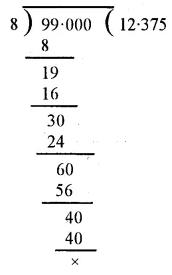Question 27.
Solution:
$$2 \frac { 19 }{ 40 }$$
= $$\\ \frac { 2\times 40+19 }{ 40 }$$
= $$\\ \frac { 80+19 }{ 40 }$$
= $$\\ \frac { 99 }{ 40 }$$
= 2.475Question 28.
Solution:
$$\\ \frac { 19 }{ 20 }$$
= 0.95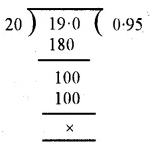Question 29.
Solution:
$$\\ \frac { 37 }{ 50 }$$
= 0.74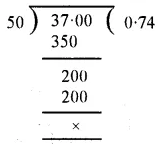Question 30.
Solution:
$$\\ \frac { 107 }{ 250 }$$
= 0.428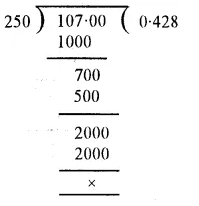Question 31.
Solution:
$$\\ \frac { 3 }{ 40 }$$
= 0.075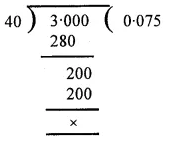Question 32.
Solution:
$$\\ \frac { 7 }{ 8 }$$
= 0.875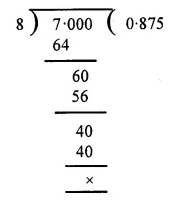Question 33.
Solution:
(i) 8 kg 640 g in kilograms
= $$8 \frac { 640 }{ 1000 }$$ kg
= 8.640kg
(ii) 9 kg 37 g in kilograms
= $$9 \frac { 37 }{ 1000 }$$ kg
= 9.037 kg.
(iii) 6 kg 8 g in kilograms
= $$6 \frac { 8 }{ 1000 }$$ kg
= 6.008 kg Ans.

Question 34.
Solution:
(i) 4 km 365 m in kilometres
= $$4 \frac { 365 }{ 1000 }$$ km
= 4.365 km
(ii) 5 km 87 m in kilometres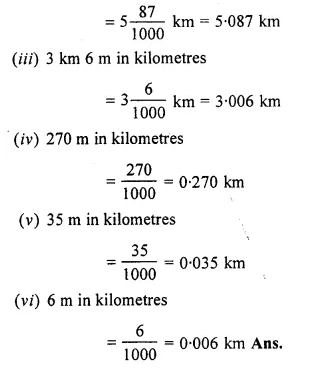Question 35.
Solution:
(i) 15 kg 850 g in kilograms
= $$15 \frac { 850 }{ 1000 }$$ kg
= 15.850 kgQuestion 36.
Solution:
(i) Rs. 18 and 25 paise in rupees
= $$18 \frac { 25 }{ 100 }$$
= 18.25 rupees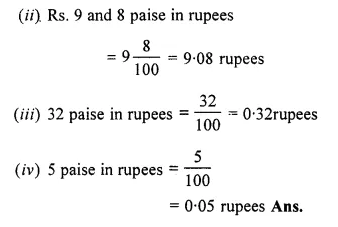Hope given RS Aggarwal Solutions Class 6 Chapter 7 Decimals Ex 7B are helpful to complete your math homework.

If you have any doubts, please comment below. Learn Insta try to provide online math tutoring for you.

## RS Aggarwal Class 6 Solutions Chapter 7 Decimals Ex 7A

These Solutions are part of RS Aggarwal Solutions Class 6. Here we have given RS Aggarwal Solutions Class 6 Chapter 7 Decimals Ex 7A.

Other Exercises

Question 1.
Solution:
(i)Fifty eight point six three = 58.63
(ii)One hundred twenty four point four two five = 124.425
(iii)Seven point seven six = 7.76
(iv)Nineteen point eight = 19.8
(v)Four hundred four point zero four four = 404.044
(vi)Point one seven three = 173
(v)Point zero one five = .015 Ans.

Question 2.
Solution:
(i) 14.83
Place value of 1 = 10,
Place value of 4 = 4,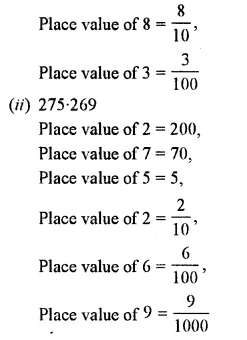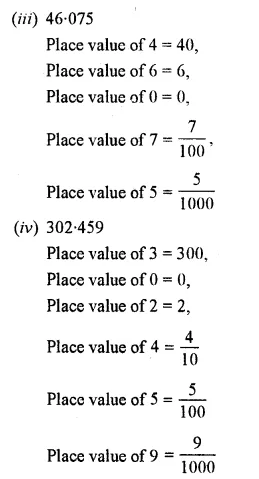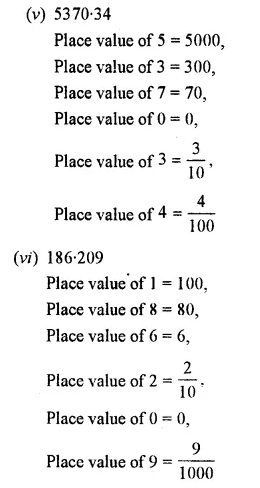Question 3.
Solution:
(i) 67.83 = (6 x 10) + (7 x 1)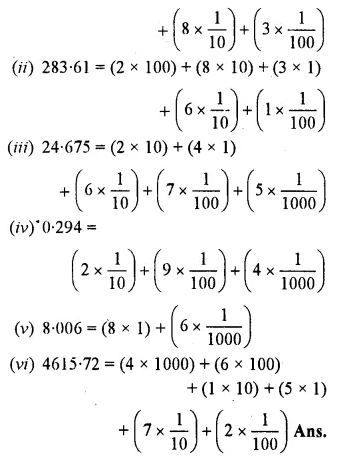Question 4.
Solution: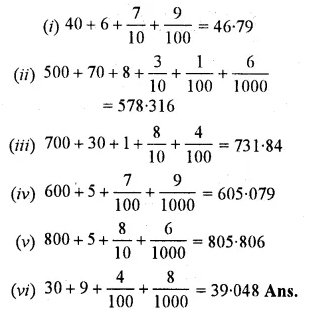Question 5.
Solution:
(i) 7.5, 64.23, 0.074 = 7.500, 64.230, 0.074
(Here, at the most 0.074 has 3 places)
(ii) 0.6, 5.937, 2.36, 4.2 = 0.600, 5.937, 2.360, 4.200
(Here, 5.937 has at most 3 places)
(iii) 1.6, 0.07, 3.58, 2.9 = 1.60, 0.07, 3.58, 2. 90
(Here, at the most are two places)
(iv) 2.5. 0.63, 14.08, 1.637 = 2.500, 0.630. 14.080, 1.637 Ans.
(Here, at the most are three places)

Question 6.
Solution:
Making like decimals where ever it is necessary,Question 7.
Solution:
First of all making them in like decimals,
(i) 5.8, 7.2, 5.69, 7.14, 5.06
=> 5.80, 7.20, 5.69, 7.14, 5.06
Arranging in ascending order,
5:06 <5.69 <5.80 <7.14 <7.20
=> 5.06 < 5.69 < 5.8 < 7.14 < 7.2 Ans.
(ii) 0.6, 6.6, 6.06, 66.6, 0.06
=>0.60, 6.60, 6.06, 66.60, 0.06
Arranging in ascending order,
0.06 < 0.60 < 6.06 < 6.60 < 66.60
=> 0.06 < 0.6 < 6.06 < 6.6 < 66.6 Ans.
(iii) 6.54, 6.45, 6.4, 6.5, 6.05
=> 6.54, 6.45, 6.4, 6.5, 6.05
Arranging in ascending order,
6. 05 < 6.40 < 6.45 < 6.50 < 6.54
=> 6.05 < 6.4 < 6.45 < 6.5 < 6.54 Ans.
(iv) 3.3,3.303, 3.033, 0.33, 3.003
=> 3.300, 3.303, 3.033, 0.330, 3.003
Arranging in descending order,
0.330 < 3.003 < 3.033 < 3.300 < 3.303
=> 0.33 < 3.003 < 3.033 < 3.3 < 3.303 Ans.

Question 8.
Solution:
Making them in like decimals and them comparing
(i) 7.3, 8.73, 73.03, 7.33, 8.073
=> 7.300, 8.730, 73.030, 7.330, 8.073
Arranging in descending order
73.030 > 8.730 > 8.073 > 7.330 > 7.300
=> 73.03 > 8.73 > 8.073 > 7.33 > 7.3 Ans.
(ii) 3.3, 3.03, 30.3, 30.03, 3.003
=> 3.300, 3.030, 30.300, 30.030, 3.003
Arranging in descending order
30.300> 30.030 >3.300 >3.030 > 3.003
=> 30.3 > 30.03 > 3.3 > 3.03 > 3.003 Ans.
(iii) 2.7, 7.2, 2.27, 2.72, 2.02, 2.007
=> 2.700, 7.200, 2.270, 2.720, 2.020, 2.007
Arranging in descending order
7. 200 > 2.720 > 2.700 > 2.270 > 2.020 > 2.007
=> 7.2 > 2.72 > 2.7 > 2.27 > 2.02 > 2.007 Ans.
(iv) 8.88, 8.088, 88.8, 88.08, ,8.008
=> 8.880, 8.088, 88.800, 88.080, 8.008
Arranging in descending order,
88.800 > 88.080 > 8.880 > 8.088 > 8.008
=> 88.8 > 88.08 > 8.88 > 8.088 > 8.008

Hope given RS Aggarwal Solutions Class 6 Chapter 7 Decimals Ex 7A are helpful to complete your math homework.

If you have any doubts, please comment below. Learn Insta try to provide online math tutoring for you.

## RS Aggarwal Class 6 Solutions Chapter 6 Simplification Ex 6B

These Solutions are part of RS Aggarwal Solutions Class 6. Here we have given RS Aggarwal Solutions Class 6 Chapter 6 Simplification Ex 6B.

Other Exercises

OBJECTIVE QUESTIONS
Mark against the correct answer in each of the following :

Question 1.
Solution:
(c) 8 + 4 ÷ 2 x 5
= 8 + 4 x $$\\ \frac { 1 }{ 2 }$$ x 5
= 8 + 10
= 18.

Question 2.
Solution:
(b) 54 ÷ 3 of 6 + 9 = 54 ÷ 18 + 9
= 54 x $$\\ \frac { 1 }{ 18 }$$ + 9
= 3 + 9
= 12.

Question 3.
Solution:
(b) 13 – (12 – 6 ÷ 3)
= 13 – (12 – 2)
= 13 – 10
= 3

Question 4.
Solution:
(a) 1001 ÷ 11 of 13
= 1001 ÷ 143
= 1001 x $$\\ \frac { 1 }{ 143 }$$
= 7.

Question 5.
Solution:
(b) 133 + 28 ÷ 7 – 8 x 2
= 133 + 4 – 16
= 137 – 16
= 121.

Question 6.
Solution:
(a) 3640 – 14 ÷ 7 x 2
= 3640 – 2 x 2
= 3640 – 4
= 3636.

Question 7.
Solution:
(b) 100 x 10 – 100 + 2000 ÷ 100
= 1000 – 100 + 20
= 920.

Question 8.
Solution:
(b) $$27-\left[ 18-\left\{ 16-\left( 5-\overline { 4-1 } \right) \right\} \right]$$
= 27 – [18 – {16 – (5 – 4 + 1)}]
= 27 – [18 – {16 – 5 + 4 – 1}]
= 27 – [18 – 16 + 5 – 4 + 1]
= 27 – 18 + 16 – 5 + 4 – 1
= 23

Question 9.
Solution:
$$32-\left[ 48\div \left\{ 36-\left( 27-\overline { 16-9 } \right) \right\} \right]$$
= 32 – [48 ÷ {36 – (27 – 16 + 9)}]
= 32 – [48 ÷ {36 – 27 + 16 – 9}]
= 32 – [48 ÷ 16]
= 32 – 3
= 29

Question 10.
Solution:
(a) 8 – [28 ÷ {34 – (36 – 18 ÷ 9 x 8)}]
$$8-\left[ 28\div \left\{ 34-\left( 36-18\times \frac { 1 }{ 9 } \times 8 \right) \right\} \right]$$
= 8 – [28 ÷ {34 – (36 – 16)}]
= 8 – [28 ÷ {34 – 20}]
= 8 – {28 ÷ 14}
= 8 – 2
= 6.

Hope given RS Aggarwal Solutions Class 6 Chapter 6 Simplification Ex 6B are helpful to complete your math homework.

If you have any doubts, please comment below. Learn Insta try to provide online math tutoring for you.

## RS Aggarwal Class 6 Solutions Chapter 5 Fractions Ex 5G

These Solutions are part of RS Aggarwal Solutions Class 6. Here we have given RS Aggarwal Solutions Class 6 Chapter 5 Fractions Ex 5G.

Other Exercises

Objective Questions :
Tick the correct answer in each of the following :

Question 1.
Solution:
(c) ∴ canceling the common factor 2, we get $$\\ \frac { 3 }{ 5 }$$

Question 2.
Solution:
(c) ∴ multiplying numerator and denominator by 4, we get $$\\ \frac { 8 }{ 12 }$$

Question 3.
Solution: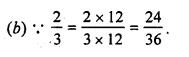Question 4.
Solution: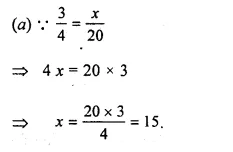Question 5.
Solution: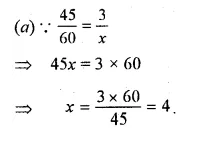Question 6.
Solution:
(c) each of the fractions has the same denominator.

Question 7.
Solution:
(d) none of these has greater denominator than its numerator.

Question 8.
Solution:
(a) its denominator is greater than its numerator.

Question 9.
Solution:
(b) their numerators are same and 4 < 5 , $$\frac { 3 }{ 4 } >\frac { 3 }{ 5 }$$

Question 10.
Solution: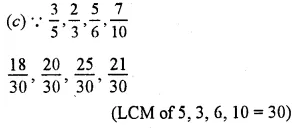Question 11.
Solution:
(b) In $$\frac { 4 }{ 5 } ,\frac { 2 }{ 7 } ,\frac { 4 }{ 9 } ,\frac { 4 }{ 11 }$$ numerator is same then the smallest denominator’s fraction is greater.

Question 12.
Solution:
(a) Denominators are same, then fraction of smallest numerator will be smallest.

Question 13.
Solution: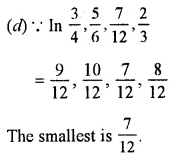Question 14.
Solution:Question 15.
Solution:Question 16.
Solution: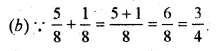Question 17.
Solution: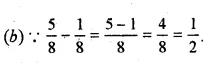Question 18.
Solution: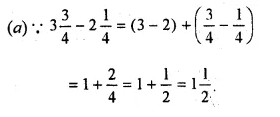Question 19.
Solution: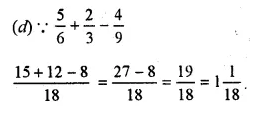Question 20.
Solution: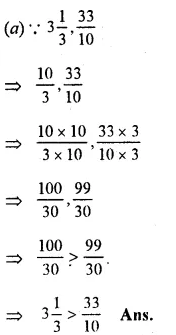Hope given RS Aggarwal Solutions Class 6 Chapter 5 Fractions Ex 5G are helpful to complete your math homework.

If you have any doubts, please comment below. Learn Insta try to provide online math tutoring for you.